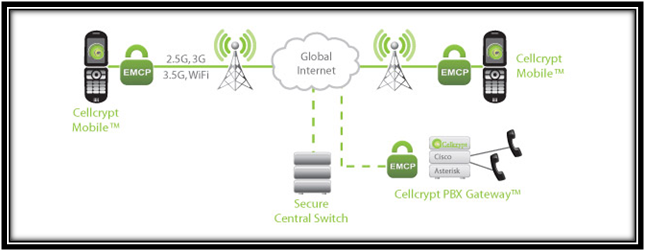# Ns2 code for RSA Algorithm

Learn about how to Implement Ns2 code for RSA Algorithm?

## what is RSA ? How RSA Algorithm is coded using NS2 Framework?

RSA encryption is a public-key encryption technology developed by RSA Data Security.RSA algorithm is based on the difficulty in factoring very large numbers.RSA encryption technique uses prime factorization as the trap door for encryption policy.Deducing an key in RSA Algorithm,  takes a huge amount of processing power and time.### How to Generate Key using RSA Algorithm?

• Generate two different primes k and y
• Calculate the modulus a = k × y
• Calculate the totient φ(n) = (k − 1) × (y− 1)
• Select for public exponent an integer e such that 1 < e < φ(a) and gcd(φ(a), e) = 1
• Calculate for the private exponent a value for d such that d = e −1 mod φ(n)
• Public Key = [e, a]
• Private Key = [d, a]

#### NS2 Projects Video Output

See our Latest Video Output of Ns2 Projects on Various Domain.

#### Ns2 Projects

Customized NS2 Projects for B.E/B.Tech/M.E/M.Tech/Ms/PhD Scholars.

#### Ns2 Projects Screen Shots

Ns2 Projects Screen Shots.Regular Update of NS2 Projects Screenshots here!

Sample code for rsa algorithm:

This is the sample code for decryption process involved in rsa algorithm.

proc rsa_slow_decrypt {
input pkey
} {

upvar \$pkey key

if {[bitsize \$key(n)] &lt; [bitsize \$input] } {

error "keysize [bitsize \$key(n)] must be greater than text [bitsize \$input]/\$input"

}
if {[catch {
set ptext [powm \$input \$key(d) \$key(n)] }] } {

puts "rsa_slow_decrypt: \$input [hex \$key(d)] [hex \$key(n)]"
error "powm error"

}
return \$ptext

}

proc pack_text {
ptext keylen
} {

# pack ptext with md5
while {[string length \$ptext] &lt; (\$keylen - 16)
} {

append ptext [binary format H* [::md5::md5 \$ptext]]

}

if {[string length \$ptext] &lt; \$keylen
} {

set md5 [binary format H* [::md5::md5 \$ptext]] append ptext [string range \$md5 0 [expr \$keylen - [string length \$ptext] - 1]]

}

# convert the string to a hex number
binary scan \$ptext H* hex
return [convert 0x\$hex] }

##### Journal Support for Research Scholars###### Ns2 Projects Work Progress
• MANET – Mobile Ad Hoc Network 95%
• VANET – Vechicle Ad Hoc Netwok 97%
• LTE – Long Term Evolution 78%
• IoT – Internet of Things 90%
• Wireless Sensor Network 89%
• Network Security 89%
• Ns2 Attacks 96%